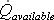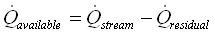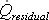# HOMER Pro 3.11

 Navigation: Glossary Flow Rate Available To Hydro TurbineType: Intermediate Variable Units: m3/s Symbol:The flow rate available to the hydro turbine is the maximum flow rate that could be diverted into the hydro turbine. In each time step, HOMER calculates the available flow rate using the following equation:where:= the total stream flow [m3/s]3/s]

HOMER uses the available stream flow to calculate the actual stream flow through the hydro turbine in each time step.

See also

Hydro Turbine Flow Rate

How HOMER Calculates the Hydro Power Output